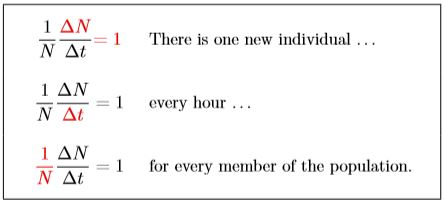# 4.1: Density-independent growth

$$\newcommand{\vecs}{\overset { \rightharpoonup} {\mathbf{#1}} }$$ $$\newcommand{\vecd}{\overset{-\!-\!\rightharpoonup}{\vphantom{a}\smash {#1}}}$$$$\newcommand{\id}{\mathrm{id}}$$ $$\newcommand{\Span}{\mathrm{span}}$$ $$\newcommand{\kernel}{\mathrm{null}\,}$$ $$\newcommand{\range}{\mathrm{range}\,}$$ $$\newcommand{\RealPart}{\mathrm{Re}}$$ $$\newcommand{\ImaginaryPart}{\mathrm{Im}}$$ $$\newcommand{\Argument}{\mathrm{Arg}}$$ $$\newcommand{\norm}{\| #1 \|}$$ $$\newcommand{\inner}{\langle #1, #2 \rangle}$$ $$\newcommand{\Span}{\mathrm{span}}$$ $$\newcommand{\id}{\mathrm{id}}$$ $$\newcommand{\Span}{\mathrm{span}}$$ $$\newcommand{\kernel}{\mathrm{null}\,}$$ $$\newcommand{\range}{\mathrm{range}\,}$$ $$\newcommand{\RealPart}{\mathrm{Re}}$$ $$\newcommand{\ImaginaryPart}{\mathrm{Im}}$$ $$\newcommand{\Argument}{\mathrm{Arg}}$$ $$\newcommand{\norm}{\| #1 \|}$$ $$\newcommand{\inner}{\langle #1, #2 \rangle}$$ $$\newcommand{\Span}{\mathrm{span}}$$

Density, in the sense of population density, refers to how many individuals are present on average per unit area. One could say, “The density of elk in Yellowstone National Park during the summer is about 3 to 6 per square mile.” Sometimes, however, you will see density used as the total number in a place. You may see, “The density of elk in Yellowstone National Park during the summer is about 10 to 20 thousand.” The symbol N is often used for population density.

In the first case above, you would write N = 4.5, the midpoint between 3 and 6. In the second case you would write N = 15000. It should be clear from context what the area is.

With this in mind, all of the following statements are equivalent:

1. The population doubles each hour. (As in the bacterial example of the previous chapter.)
2. The population N(t) doubles each hour. Here the number of individuals is represented by the letter N, and N(t) means population at time t. In the bacterial example, there was one bacterium at the beginning of the experiment when the clock started running, so you would write N (0) = 1. One hour later, the hypothetical population had doubled, so you would write N (1) = 2. Doubling successively then gives N (2) = 4, N (3) = 8, and so forth until after five days, or 120 hours, N (120) = 1036, or slightly more—enough to fill all the oceans of the world.
3. The population N doubles each hour. Often the “(t )” is left off for simplicity, it being understood that the population N is a function of time.
4. N doubles each hour. Since N represents a population in this case, the word “population“ will often be dropped for conciseness.
5. N (t + 1) = 2N (t ). In English this would be read “N of t plus 1 equals two times N of t.” That simply means that the population at some time, anytime, t, when multiplied by 2, is the population in the next time step, t plus one.
6. The change in the population each hour is equal to the size of the population that hour. This may sound pretty confusing. But it means that the amount that the population increases in the time step is equal in size to the whole population. Usually the increase is much less than that, perhaps a few percent, but here we are dealing with a rapidly increasing bacterial population.
7. The change in the population each hour is N (t +1) minus N(t), which is to say N (t +1)− N (t ) = 2N (t )−N (t ) = N (t ). Here the population in the next hour, N (t +1), minus the population now, N (t ), which is the change in population, is twice the current population, 2N (t ), minus the current population, N (t ) (not less confusing, perhaps).
8. The change in the population each hour, call it “Delta N” or ∆N, is ∆N /∆t = 2N(t)N(t) = N(t). Here the symbol delta (∆) means change in or the difference. So ∆N means the change in N, and ∆t means the change in t. So the change in N per unit of time is written ∆N /∆t, where delta t is the time unit being used, such as hour or day. This statement is thus the same as the previous one, but with symbols to shorten it.
9. N /∆t = N. This means the population change in each time unit is equal to the population size itself. That is just because the population is doubling each time step.
10. $$\frac{1}{N}\, \,frac{∆N}{∆t}\, =\,1$$ This is just dividing both sides of the previous equation by N, and perhaps looks even more confusing. However, in what follows, it turns out to be the most useful of all.

To move forward, let’s focus on the last equation, with its parts colored in the box below.In the first row, the “∆N = 1” refers to a change in the population of one individual, because delta (∆) means change. In the second row, the “∆t ” in the denominator modifies this to the change in each time step—in this, case each hour. In the third row, the 1/N modifies it drastically to mean the change in the population per individual in the population.

This could mean that one new individual is born while the parent lives on, or that two new individuals are born and the parent dies, or that the parent divides in two, or other equivalent events. In this model, these details are abstractions that do not matter for purposes of projecting the population. The model simply records the number of offspring produced by each member of the population and surviving to reproduce. Multiplied by 100, this becomes the percentage growth of the population. For humans, this is like the number of children per family who survive to adulthood. (Though it has to be divided by two if there are two parents per family.) You have seen how rapidly that blows up, from the calculation in Chapter 3.Figure $$\PageIndex{1}$$. Wild Rudbeckia hirta with an unusual stroke of red boldly bisecting its petals.

This page titled 4.1: Density-independent growth is shared under a CC BY-NC 4.0 license and was authored, remixed, and/or curated by Clarence Lehman, Shelby Loberg, & Adam Clark (University of Minnesota Libraries Publishing) via source content that was edited to the style and standards of the LibreTexts platform; a detailed edit history is available upon request.